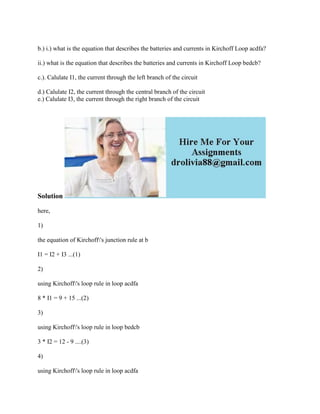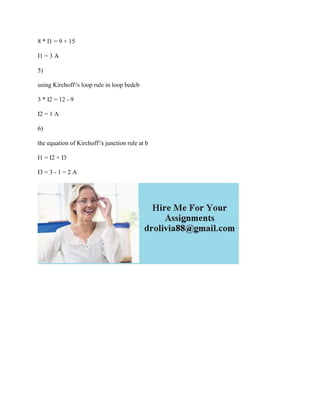Diese Präsentation wurde erfolgreich gemeldet.

# b-) i-) what is the equation that describes the batteries and currents.docx

Anzeige
Anzeige
Anzeige
Anzeige
Anzeige
Anzeige
Anzeige
Anzeige
Anzeige
Anzeige
Anzeige×

1 von 2 Anzeige

# b-) i-) what is the equation that describes the batteries and currents.docx

b.) i.) what is the equation that describes the batteries and currents in Kirchoff Loop acdfa?

ii.) what is the equation that describes the batteries and currents in Kirchoff Loop bedcb?

c.). Calulate I1, the current through the left branch of the circuit

d.) Calulate I2, the current through the central branch of the circuit
e.) Calulate I3, the current through the right branch of the circuit

Solution
here,
1)
the equation of Kirchoff\'s junction rule at b
I1 = I2 + I3 ...(1)
2)
using Kirchoff\'s loop rule in loop acdfa
8 * I1 = 9 + 15 ...(2)
3)
using Kirchoff\'s loop rule in loop bedcb
3 * I2 = 12 - 9 ....(3)
4)
using Kirchoff\'s loop rule in loop acdfa
8 * I1 = 9 + 15
I1 = 3 A
5)
using Kirchoff\'s loop rule in loop bedcb
3 * I2 = 12 - 9
I2 = 1 A
6)
the equation of Kirchoff\'s junction rule at b
I1 = I2 + I3
I3 = 3 - 1 = 2 A
.

b.) i.) what is the equation that describes the batteries and currents in Kirchoff Loop acdfa?

ii.) what is the equation that describes the batteries and currents in Kirchoff Loop bedcb?

c.). Calulate I1, the current through the left branch of the circuit

d.) Calulate I2, the current through the central branch of the circuit
e.) Calulate I3, the current through the right branch of the circuit

Solution
here,
1)
the equation of Kirchoff\'s junction rule at b
I1 = I2 + I3 ...(1)
2)
using Kirchoff\'s loop rule in loop acdfa
8 * I1 = 9 + 15 ...(2)
3)
using Kirchoff\'s loop rule in loop bedcb
3 * I2 = 12 - 9 ....(3)
4)
using Kirchoff\'s loop rule in loop acdfa
8 * I1 = 9 + 15
I1 = 3 A
5)
using Kirchoff\'s loop rule in loop bedcb
3 * I2 = 12 - 9
I2 = 1 A
6)
the equation of Kirchoff\'s junction rule at b
I1 = I2 + I3
I3 = 3 - 1 = 2 A
.

Anzeige
Anzeige

### b-) i-) what is the equation that describes the batteries and currents.docx

1. 1. b.) i.) what is the equation that describes the batteries and currents in Kirchoff Loop acdfa? ii.) what is the equation that describes the batteries and currents in Kirchoff Loop bedcb? c.). Calulate I1, the current through the left branch of the circuit d.) Calulate I2, the current through the central branch of the circuit e.) Calulate I3, the current through the right branch of the circuit Solution here, 1) the equation of Kirchoff's junction rule at b I1 = I2 + I3 ...(1) 2) using Kirchoff's loop rule in loop acdfa 8 * I1 = 9 + 15 ...(2) 3) using Kirchoff's loop rule in loop bedcb 3 * I2 = 12 - 9 ....(3) 4) using Kirchoff's loop rule in loop acdfa
2. 2. 8 * I1 = 9 + 15 I1 = 3 A 5) using Kirchoff's loop rule in loop bedcb 3 * I2 = 12 - 9 I2 = 1 A 6) the equation of Kirchoff's junction rule at b I1 = I2 + I3 I3 = 3 - 1 = 2 A